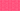PricingResources

# Black Scholes definition and equation

Written by

If you’re interested in buying and selling options contracts, the Black Scholes formula is a useful equation to have on hand. Learn more about how the Black Scholes model works, as well as when it’s best applied in trading.

## What is the Black Scholes model?

The Black Scholes model is used to determine a fair price for an options contract. This mathematical equation can estimate how financial instruments like future contracts and stock shares will vary in price over time.

There are several variables that go into the Black Scholes formula, including:

• Volatility

• Price of underlying asset

• Strike price

• Time until expiration

• Risk-free interest rate

By plugging these variables into the equation, sellers can set realistic prices for their options contracts.

The formula was developed by economists Fischer Black, Myron Scholes and Robert Merton, which is why it’s also called the Black Scholes Merton formula. Initially published in the Journal of Political Economy in 1973, the Black Scholes model went on to win its developers the Nobel Prize.

## How does the Black Scholes model work?

Based on the idea that heavily traded financial instruments come with inherent volatility and drift, the formula is designed to reflect consistent price variation. For stocks, it also incorporates the time value of money.

No matter the contract it’s applied to, the model makes several assumptions. These include the following:

• The option only works with European options that come with a firm expiration date.

• Markets are efficient and liquid.

• No transaction costs are included.

• The volatility and risk-free rate of the underlying asset are both constant.

• The underlying asset’s returns follow a lognormal distribution.

The model works with the assumption that asset prices can’t be negative, because zero is used as a boundary. This is why stock prices must follow a normal pattern of distribution.

Normally, the Black Scholes model also assumes that no dividends are paid out during the option’s lifespan. However, it’s possible to adapt the equation for dividends by factoring in the ex-dividend date value.

## The Black Scholes equation

Options traders tend to use online a Black Scholes calculator or trading platforms that automatically work out the equation, because it’s quite complicated written out on paper. Trading platforms also offer additional benefits like analysis tools and spreadsheets to clearly lay out all options pricing.

If you’re numbers-savvy, you can work out the formula yourself with the following Black Scholes equation.

In the Black Scholes formula, the variables are as follows:

• C = price of the call option or theoretical option value

• S = current stock price

• E = exercise price in the option contract

• R = risk-free interest rate

• T = time in years until expiration date

• σ = measure of volatility of the underlying asset

• N(d) = the probability that a value less than (d) occurs

• ert = discount factor

• ln = natural logarithm

Essentially, first you multiply your stock price (or other underlying asset price) by the cumulative standard normal probability. After this, the net present value of the strike price is multiplied by the cumulative standard normal distribution. This is subtracted from the result of the first calculation.

It’s easier to simply plug these numbers into a Black Scholes calculator rather than doing the complicated maths yourself. No matter how you arrive at the results, they represent the current value of the call option according to the likely stock price at the time of expiration. This helps you set a fair price as a call options seller.

## Limitations of the Black Scholes formula

The Black Scholes model is useful on many occasions, but it does have its limitations to be aware of. To begin with, it’s only applicable to European options because U.S. call options can be exercised before the contract’s expiration date. It doesn’t take transaction fees or taxes into account, nor does the original model factor in dividends.

It also makes assumptions that risk-free rates and volatility are constant over time, which doesn’t reflect real life. Volatility can fluctuate according to supply and demand, so you should keep all of these limits in mind as you work out your price.

## We can help

GoCardless helps you automate payment collection, cutting down on the amount of admin your team needs to deal with when chasing invoices. Find out how GoCardless can help you with ad hoc payments or recurring payments.

Sales

Support

+61 3 8375 9198